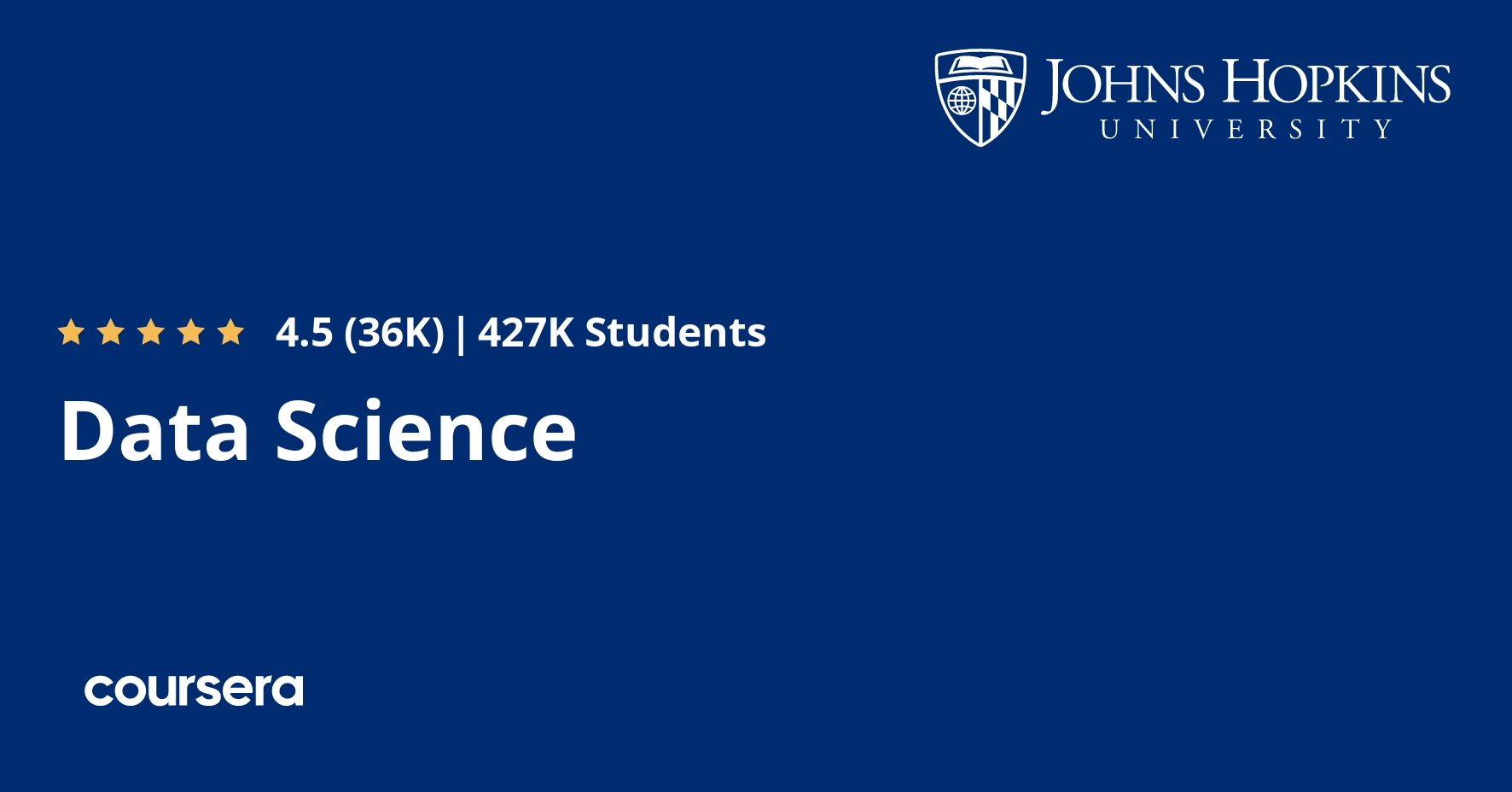# Data Science Specialization## What you will learn from the Data Science Specialization Course

• Set up R, R-Studio, Github and other useful tools
• Understand the data, problems, and tools that data analysts use
• Explain essential study design concepts
• Create a Github repository
• Learn about version control and why it’s so important to data scientists
• How to use Git and GitHub to manage version control in data science projects
• Learn to use R Markdown
• How to program in R and how to use R for effective data analysis
• Understand critical programming language concepts
• Configure statistical programming software
• Make use of R loop functions and debugging tools
• Collect detailed information using R profiler
• Programming with R
• Loop functions and the debugging tools in R
• How to simulate data in R and most useful function in R
• Understand common data storage systems
• Apply data cleaning basics to make data “tidy”
• Use R for text and date manipulation
• Obtain usable data from the web, APIs, and databases
• Finding data and reading different file types
• Organizing, merging, and managing the data from the web or from databases like MySQL
• Text and date manipulation in R
• Understand analytic graphics and the base plotting system in R
• Use advanced graphing systems such as the Lattice system
• Make graphical displays of very high dimensional data
• Apply cluster analysis techniques to locate patterns in data
• Basics of analytic graphics and the base plotting system in R
• Advanced graphing systems such as the Lattice system and the ggplot2 system
• Cluster analysis techniques
• Organize data analysis to help make it more reproducible
• Write up a reproducible data analysis using knitr
• Determine the reproducibility of analysis project
• Publish reproducible web documents using Markdown
• Core tools for developing reproducible documents
• Basic checklist for ensuring that data analysis is reproducible
• Understand the process of drawing conclusions about populations or scientific truths from data
• Describe variability, distributions, limits, and confidence intervals
• Use p-values, confidence intervals, and permutation tests
• Make informed data analysis decisions
• Fundamentals of probability, random variables, expectations and more
• Use regression analysis, least squares and inference
• Understand ANOVA and ANCOVA model cases
• Investigate analysis of residuals and variability
• Describe novel uses of regression models such as scatterplot smoothing
• Least Squares and Linear Regression & Multivariable Regression
• Generalized linear models, including binary outcomes and Poisson regression
• Use the basic components of building and applying prediction functions
• Understand concepts such as training and tests sets, overfitting, and error rates
• Describe machine learning methods such as regression or classification trees
• Explain the complete process of building prediction functions
• Regularized Regression and Combining Predictors
• Develop basic applications and interactive graphics using GoogleVis
• Use Leaflet to create interactive annotated maps
• Build an R Markdown presentation that includes a data visualization
• Create a data product that tells a story to a mass audience
• How to develop basic applications and interactive graphics in shiny
• How to compose interactive HTML graphics with GoogleVis
• How to prepare data visualizations with Plotly
• How to create R Markdown files and embed R code in an Rmd
• How to create R packages
• Create a useful data product for the public
• Apply your exploratory data analysis skills
• Build an efficient and accurate prediction model
• Produce a presentation deck to showcase your findings

### Data Science Specialization includes 10 Courses they are

Course Instructors Jeff Leek, Roger D. Peng, Brian Caffo Offered by Johns Hopkins University Through Coursera

Note: 100% OFF Udemy coupon codes are valid for maximum 3 days only. Look for "ENROLL NOW" button at the end of the post.
Disclosure: This post may contain affiliate links and we may get small commission if you make a purchase. Read more about Read more about Affiliate disclosure here.
Deal Score0